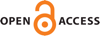Download this articleFor screen For printingRecent IssuesThe Journal About the Journal Editorial Board Editorial Interests Editorial Procedure Subscriptions Submission Guidelines Submission Page Policies for Authors Ethics Statement ISSN (electronic): 1364-0380 ISSN (print): 1465-3060 Author Index To Appear Other MSP Journals$d_p$–convergence and $\epsilon$–regularity theorems for entropy and scalar curvature lower bounds

### Man-Chun Lee, Aaron Naber and Robin Neumayer

Geometry & Topology 27 (2023) 227–350##### Abstract

Consider a sequence of Riemannian manifolds $\left({M}_{i}^{n},{g}_{i}\right)$ whose scalar curvatures and entropies are bounded from below by small constants ${R}_{i},{\mu }_{i}\ge -{𝜖}_{i}$. The goal of this paper is to understand notions of convergence and the structure of limits for such spaces. As a first issue, even in the seemingly rigid case ${𝜖}_{i}\to 0$, we will construct examples showing that from the Gromov–Hausdorff or intrinsic flat points of view, such a sequence may converge wildly, in particular to metric spaces with varying dimensions and topologies and at best a Finsler-type structure. On the other hand, we will see that these classical notions of convergence are the incorrect ones to consider. Indeed, even a metric space is the wrong underlying category to be working on.

Instead, we will introduce a weaker notion of convergence called ${d}_{p}$–convergence, which is valid for a class of rectifiable Riemannian spaces. These rectifiable spaces will have a well-behaved topology, measure theory and analysis. This includes the existence of gradients of functions and absolutely continuous curves, though potentially there will be no reasonably associated distance function. Under this ${d}_{p}$ notion of closeness, a space with almost nonnegative scalar curvature and small entropy bounds must in fact always be close to Euclidean space, and this will constitute our $𝜖$–regularity theorem. In particular, any sequence $\left({M}_{i}^{n},{g}_{i}\right)$ with lower scalar curvature and entropies tending to zero must ${d}_{p}$–converge to Euclidean space.

More generally, we have a compactness theorem saying that sequences of Riemannian manifolds $\left({M}_{i}^{n},{g}_{i}\right)$ with small lower scalar curvature and entropy bounds ${R}_{i},{\mu }_{i}\ge -𝜖$ must ${d}_{p}$–converge to such a rectifiable Riemannian space $X$. In the context of the examples from the first paragraph, it may be that the distance functions of ${M}_{i}$ are degenerating, even though in a well-defined sense the analysis cannot be. Applications for manifolds with small scalar and entropy lower bounds include an ${L}^{\infty }$–Sobolev embedding and a priori ${L}^{p}$ scalar curvature bounds for $p<1$.

##### Keywords
scalar curvature, epsilon regularity, metric spaces
Primary: 53C21
##### Publication